# Find an expression for a sequence involving the sum of nth powers

• rugerts

#### rugerts

Homework Statement
a_n = 2*a_n-1 + a_n-2 -2*a_n-3.
a_0 = 5
a_1 = 18
a_2 = 14
Relevant Equations
No real equations. Shown below is a similar example done in class that I'm trying to base my solution around.
Example done in class: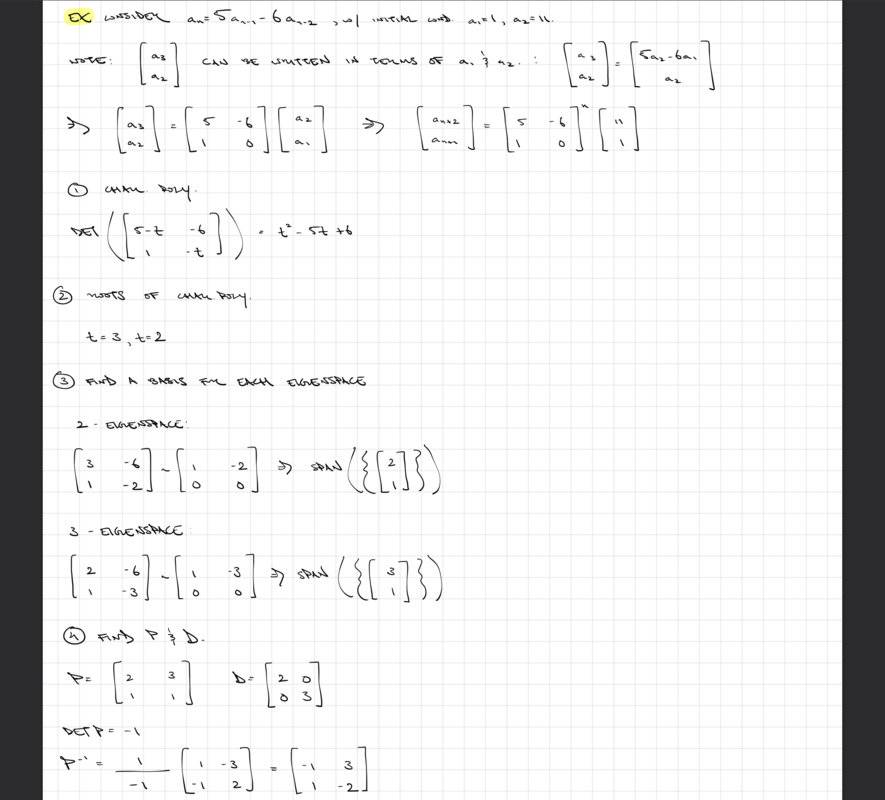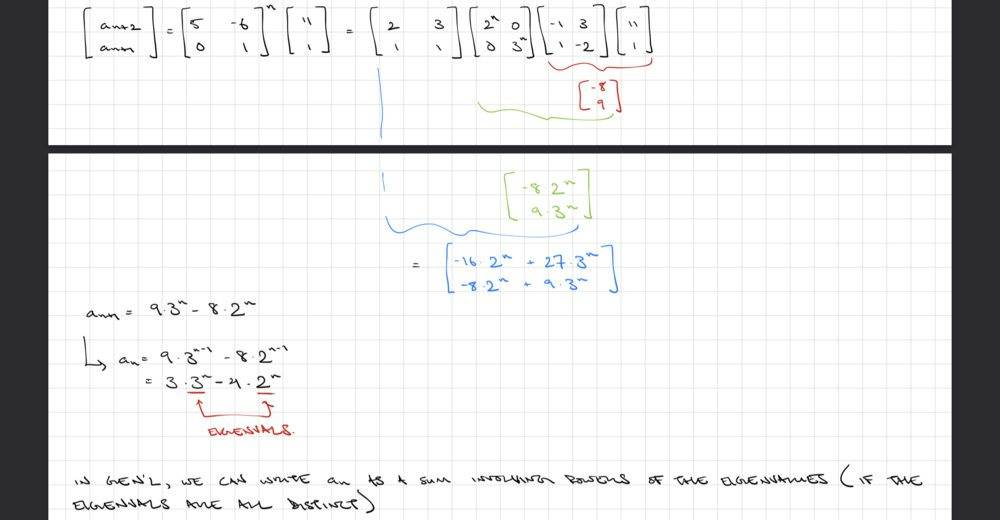The problem and my solution: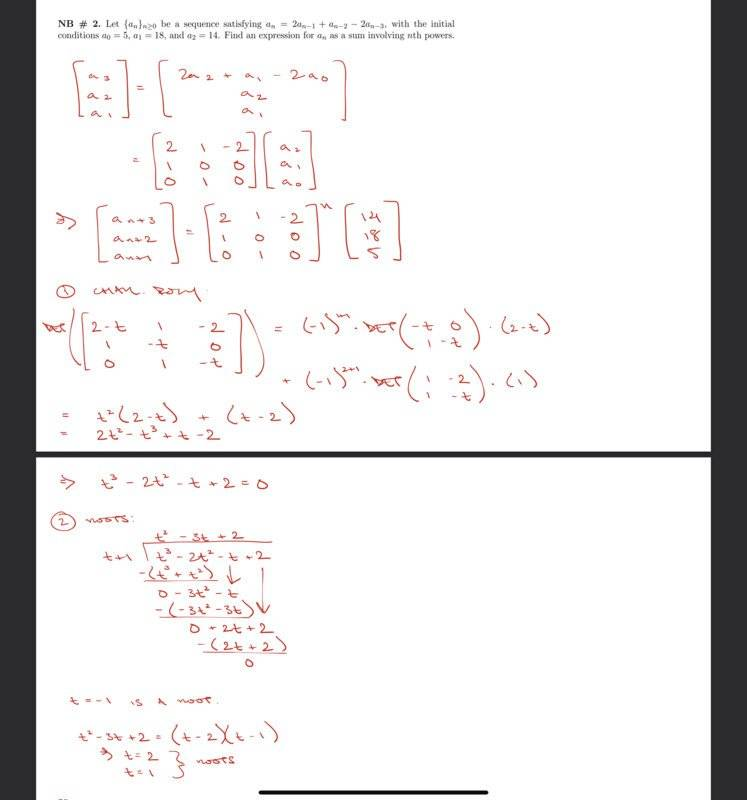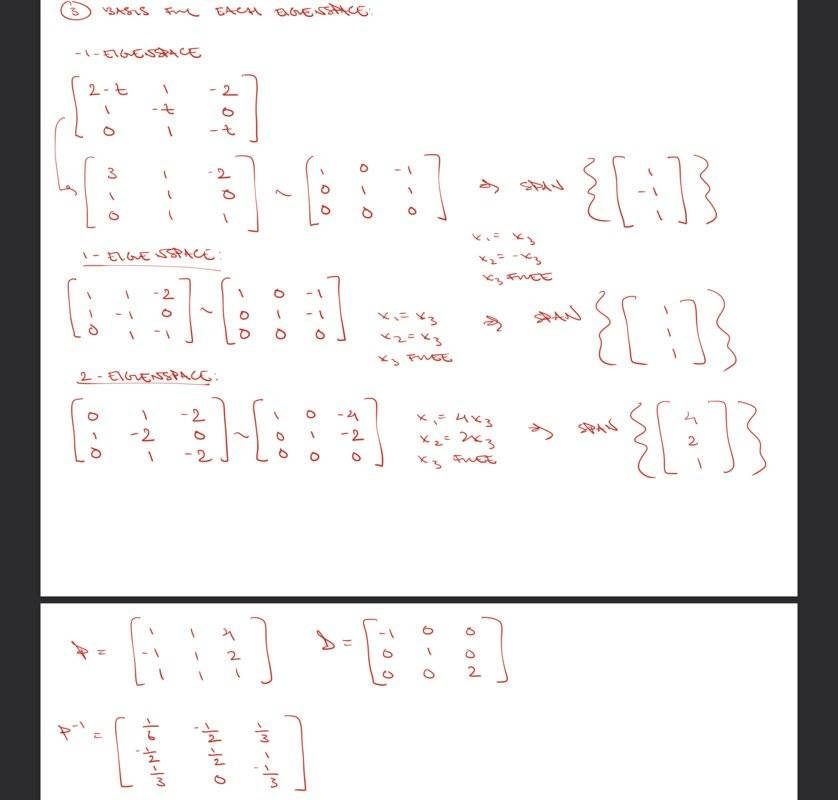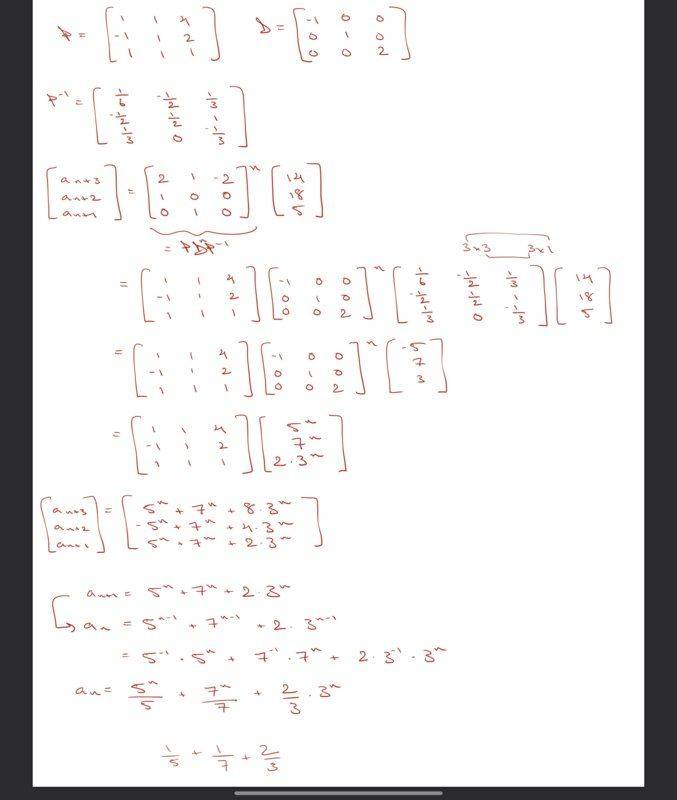My solution seems incorrect because if I try to plug in 0, I don't get the initial condition given in the problem.

Does anyone see what I've done wrong along the way?

Thanks.

#### Attachments

The equations would be much easier to read when typed here, with explanations what you do why.
$$a_n = \frac{5^n}{5} + \frac{7^n}{7} + \frac 2 3 3^n$$
You shouldn't get three different equations for an+1, an+2 and an+3.

Where do you plug in your initial conditions? You should have a general solution with three unknowns (which looks very different from what you got), these unknowns can be computed based on the initial conditions.

The equations would be much easier to read when typed here, with explanations what you do why.
$$a_n = \frac{5^n}{5} + \frac{7^n}{7} + \frac 2 3 3^n$$
You shouldn't get three different equations for an+1, an+2 and an+3.

Where do you plug in your initial conditions? You should have a general solution with three unknowns (which looks very different from what you got), these unknowns can be computed based on the initial conditions.
Did you look through the first example I was shown in lecture? That general solution only has powers of n in it, which I think is the goal. Maybe I'm misunderstanding you.

Not been through all your calculation, but I think you can do a quite simpler calculation in this case if you define a new variable, either
bn = an - 2an-1
or probably better
cn = an - an-2.

You then get a very simple series for the new variable, which you can convert into one, well maybe you should call it two, for the an .

Not been through all your calculation, but I think you can do a quite simpler calculation in this case if you define a new variable, either
bn = an - 2an-1
or probably better
cn = an - an-2.

You then get a very simple series for the new variable, which you can convert into one, well maybe you should call it two, for the an .
Interesting. I hate to turn down simpler solutions but I think, since this is a course in linear algebra and we've just covered diagonalization, that we're expected to solve this using that technique.

Did you look through the first example I was shown in lecture? That general solution only has powers of n in it, which I think is the goal. Maybe I'm misunderstanding you.
If you consider powers of 0 and -1 it will work...

So I actually had a mistake in matrix multiplication. I've got the correct solution now. My approach was fine. Thanks all.

•WWGD